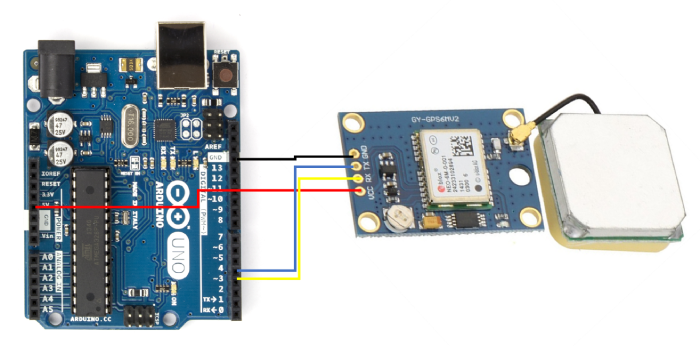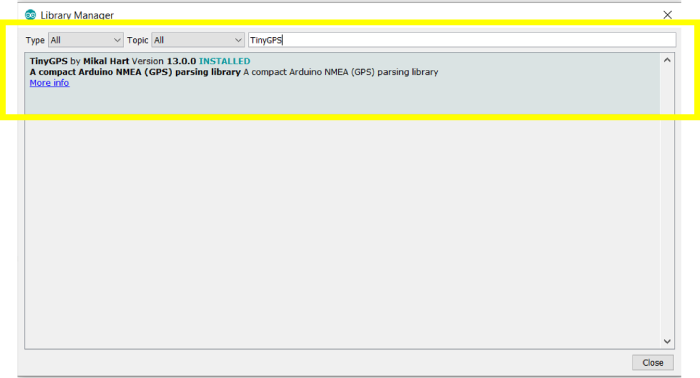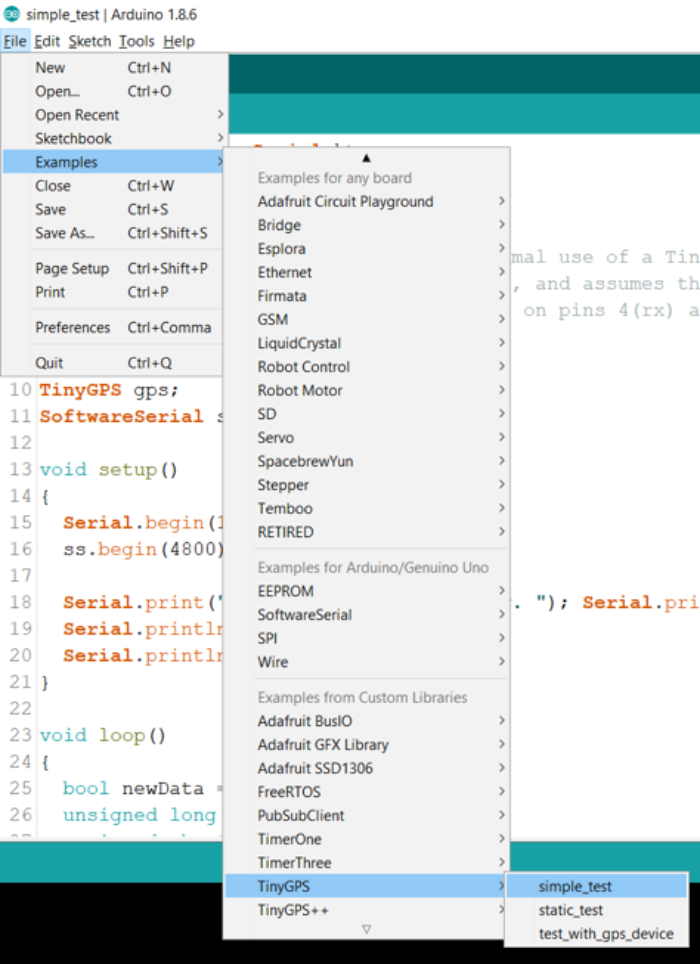# Interfacing GNSS receiver with Arduino to get speed

ArduinoArduino BoardsArduino IDEArduino Programming Language

In this tutorial, we will interface Arduino with a GNSS Receiver and obtain the speed. If possible, you can run this code in a moving vehicle, because otherwise you will get 0 speed if your GNSS receiver is stationary. Any GNSS receiver generally uses UART for communication. We will be using the ublox Neo-6M GNSS module for this

## Circuit DiagramAs you can see, we connect Vcc to 5V, GND to GND, RX of the Neo 6M to pin 3 of Arduino Uno, and TX of Neo 6M to pin 4 of Arduino Uno.

## Required Libraries

TinyGPS library will be required for interfacing Arduino Uno with the OLED Display −

Go to Tools → Manage Libraries, search for this library, and click Install.## Code Walkthrough

In a previous article, we have already seen how to obtain the latitude and longitude from the GNSS receiver using the TinyGPS library. You can also obtain that code from File →Examples → TinyGPS → simple_test.Alternatively, the code can be accessed on GitHub here − https://github.com/mikalhart/TinyGPS/blob/master/examples/simple_test/simple_test.ino

Now, we won’t walk through this code again. We will just discuss the changes required to get the speed as well.

In the loop, within the if(newData) block, in the first line, where flat, flon are defined, add another float, speed_kmph

float flat, flon, speed_kmph;

Then, at the end of this block, add the following lines −

Serial.print(" SPEED=");
Serial.print(speed_kmph == TinyGPS::GPS_INVALID_F_SPEED ? 0 :
gps.f_speed_kmph(), 2);

That’s it! Now the GPS speed will also get printed, along with the position. The speed can be printed in other units as well, using different functions −

gps.f_speed_knots(); // speed in knots
gps.f_speed_mph(); // speed in miles/hr
gps.f_speed_mps(); // speed in m/sec

Similarly, there are other functions, that give the course of the receiver in degrees, the current date and time, distance between two points, etc.

You are also encouraged to go through the other examples that come with this library.